MORE IN Design and Analysis of Algorithms
VTU Computer Science (Semester 4)
Design and Analysis of Algorithms
December 2014
Total marks: --
Total time: --
INSTRUCTIONS
(1) Assume appropriate data and state your reasons
(2) Marks are given to the right of every question
(3) Draw neat diagrams wherever necessary

1 (a) Find ged(31415, 14142) by applying Euclid's algorithm. Estimate how many times it is faster when compared to the algorithm based on consecutive integer checking.
4 M
1 (b) Compare the order growth of 1/2 n(n-1) and n2.
4 M
1 (c) Explain the mathematical analysis of Fibonacci recursive algorithm.
6 M
1 (d) Write Brute force string matching algorithm.
6 M

2 (a) Find the upper bound of recurrences given below by substitution method. $\displaystyle i) \ 2T \left ( \dfrac {n}{2} \right )+n \\ ii) \ T\left ( \dfrac {n}{2} \right )+1$
6 M
2 (b) Sort the following elements using merge sort. Write the recursion tree.
70, 20, 30, 40, 10, 50, 60
6 M
2 (c) Write the algorithm for quick sort. Derive the worst case time efficiency of the algorithm.
8 M

3 (a) Write greedy method control abstraction for subset paradigm.
4 M
3 (b) Using greedy method, trace the following graph to get shortest path from vertex 'a' to all other vertices.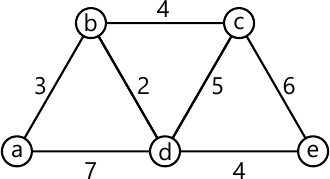6 M
3 (c) What is the solution generated by the function job scheduling (JS) when n=5,
[P1, P2, P3, P4, P5]=[20, 15, 10, 5, 1] and
[d1, d2, d3, d4, d5, = [2, 2, 1, 3, 3]
6 M
3 (d) Apply PRIMS algorithm for the following graph to find minimum spanning tree.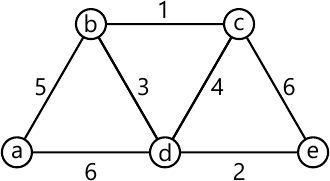4 M

4 (a) Using dynamic programming, compute the shortest path from vertex 1 to all other vertices.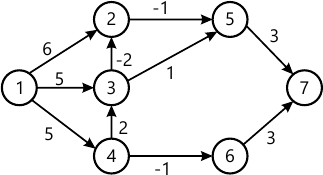10 M
4 (b) Solve the Knapsack n=3, {W1, W2, W3}={1, 2, 2} and {P1, P2, P3}={18, 16, 6} and M=4 dynamic programming.
4 M
4 (c) For the given graph, obtain optimal cost tour using dynamic programming.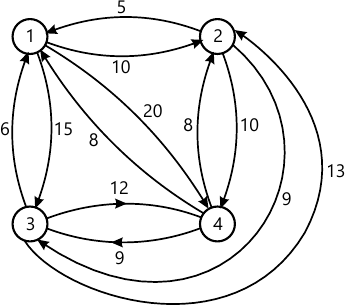6 M

5 (a) What are the three variations of decrease and conquer technique.
3 M
5 (b) Conduct DFS for the following graph.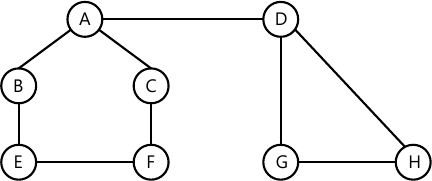5 M
5 (c) Apply DFS based algorithm to solve topological sorting problem for the following graph: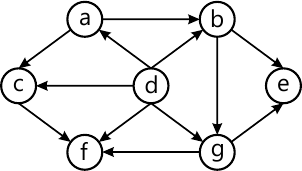6 M
5 (d) Construct shift table for the pattern EARN and search for the same in text FAIL - MEANS - FIRST - ATTEMPT - IN - LEARNING using Horspool algorithm.
6 M

6 (a) Explain the four methods used to establish lower bounds of algorithm.
8 M
6 (b) Define decision trees. Write the decision tree for the three element selection sort.
6 M
6 (c) Define P, NP and NP complete problems.
6 M

7 (a) Explain how back tracking used for solving 4-queens problem. Write the state space tree.
6 M
7 (b) Solve the following assignment problem using branch and bound method.
 Job1 Job2 Job3 Job4 Person a 9 2 7 8 Person b 6 4 3 7 Person c 5 8 1 8 Person d 7 6 9 4
8 M
7 (c) Apply twice-around-the-tree algorithm for the travelling sales person problem for the following graph.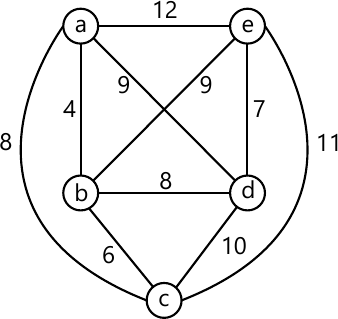6 M

8 (a) Explain the various models for parallel computations.
9 M
8 (b) Let the i/p to the prefix computations be 5, 12, 8, 6, 3, 9, 11, 12, 1, 5, 6, 7, 10, 4, 3, 5 and three are four processors and ? stands for addition. With diagram explain how prefix computation is done by parallel algorithm.
8 M
8 (c) Explain how matrix M is computed using parallel algorithm for the given graph.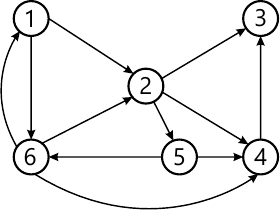3 M

More question papers from Design and Analysis of Algorithms